• ## 向量与矩阵的卷积算法

万次阅读 多人点赞 2015-02-04 00:56:44
由于我发现网上并没有关于向量与矩阵两者进行卷积计算具体算法，所以我就跟各位网友分享一下我观点。因为本人知识储备有限，对卷积的了解也很是肤浅，没有深入研究，所以有错误地方还请大神们指正，小子不胜...
由于我发现网上并没有关于向量与矩阵两者进行卷积计算的具体算法，所以我就跟各位网友分享一下我的观点。因为本人知识储备有限，对卷积的了解也很是肤浅，没有深入研究，所以有错误的地方还请大神们指正，小子不胜感激。

声明一下，以下算法是经过实例验证的，所以从数据的值的层面上来说是正确的。

在进入正题之前，需要跟大家说明一下，本篇文章主要是针对matlab中conv2( , , ‘same’);这句的翻译，对于其它格式，尚未研究。至于什么是卷积，它的数学表达式，作用等等问题，维基百科和各大神的博客上都有，这里就不再赘述。

在matlab中，函数conv和conv2都是用来计算卷积的，因为这里是对二维矩阵进行卷积计算，并且结果也是一个二维矩阵，所以用conv2。对于连续函数的卷积计算是求积分，对离散型的，例如矩阵，求卷积时，是向量的各元素值与对应位置上的矩阵的值相乘之后求和，并用所求和生成一个新的矩阵。图解如下：

设现有 4*4 矩阵f = [1 2 3 4 ; 5 6 7 8 ; 9 10 11 12 ; 13 14 15 16]，向量l = [2 3 1]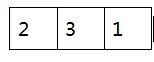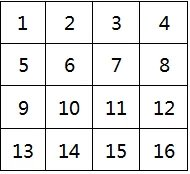1、对于没有类型参数’same’的conv2，生成的矩阵行数与原矩阵相同，列数为向量列数和矩阵列数之和减一。具体算法：

（1）先将向量反褶，此处即反褶为[1 3 2];

（2）将反褶后的向量在矩阵上一行一行滑动，并将重叠部分的两个值相乘，将所有的乘积求和，然后产生一个新的值。对第一行的计算过程如下，其余行相同。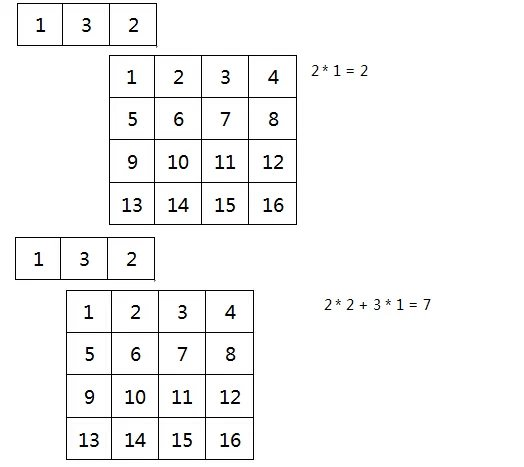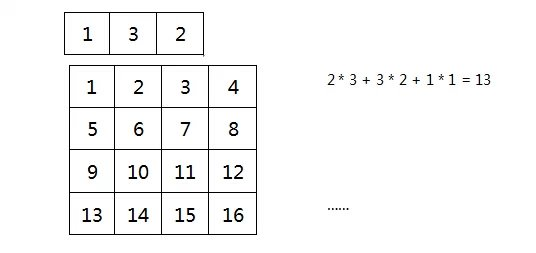计算结果如下：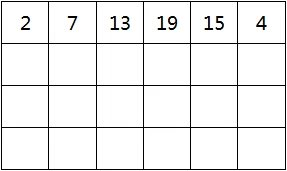2、对于有类型‘same’的conv2，所得结果是一个与原矩阵相同大小的矩阵，所以相对于上面的算法，需要舍弃一部分结果，而保存结果的方式，跟图像腐蚀的原理类似。需要有一个核，这里的核就是向量里的某个元素，用他遍历矩阵，重叠部分算法和上面一样。具体步骤如下：

（1）将向量反褶

（2）确定核，设向量的长度为ksize，那么核的在向量中的角标就是ksize - (int)((ksize + 2) / 2)。

（3）用核遍历矩阵，相乘求和。

图解如下：

角标 = 3 – 2 = 1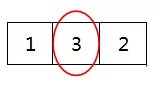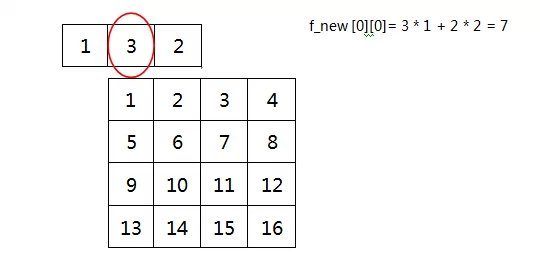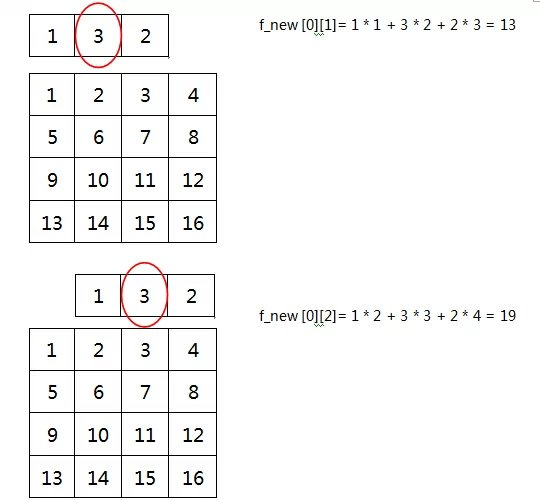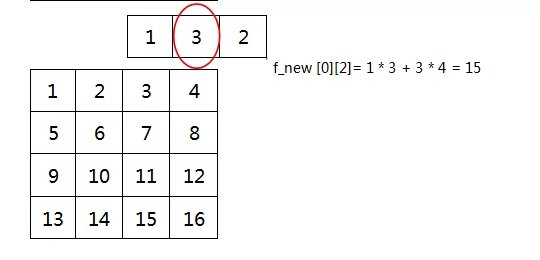结果如下：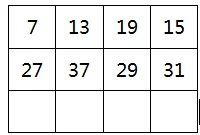以上便是我根据实际总结的算法，与matlab运行结果吻合，同理，列向量与矩阵的卷积算法相同。

小技巧：列向量与矩阵卷积：如果是用C语言写卷积函数，只需要写一个方向，即行向量与矩阵卷积即可，C语言中，向量的存储方式是线性的，所以行向量和列向量在内存上没区别，当列向量求卷积的时候，只需要将矩阵转置，让后按照行向量的求法求卷积，再把结果转置回来就行了。
展开全文matlab c语言 实例
• 主流深度学习软件在进行卷积操作时候并不是采用我们非常熟悉但是效率底下双重循环算法，而是将卷积表示为一个由卷积核导出稀疏矩阵与拉伸成一维影像向量的矩阵乘积，这样可以充分利用GPU带来并行计算收益...


主流深度学习软件在进行卷积操作的时候并不是采用我们非常熟悉但是效率底下的双重循环算法，而是将卷积操作转化为一个由卷积核导出的稀疏矩阵与拉伸成一维影像向量的矩阵乘积，只有这样，才可以充分利用GPU并行计算所带来的收益。（图1）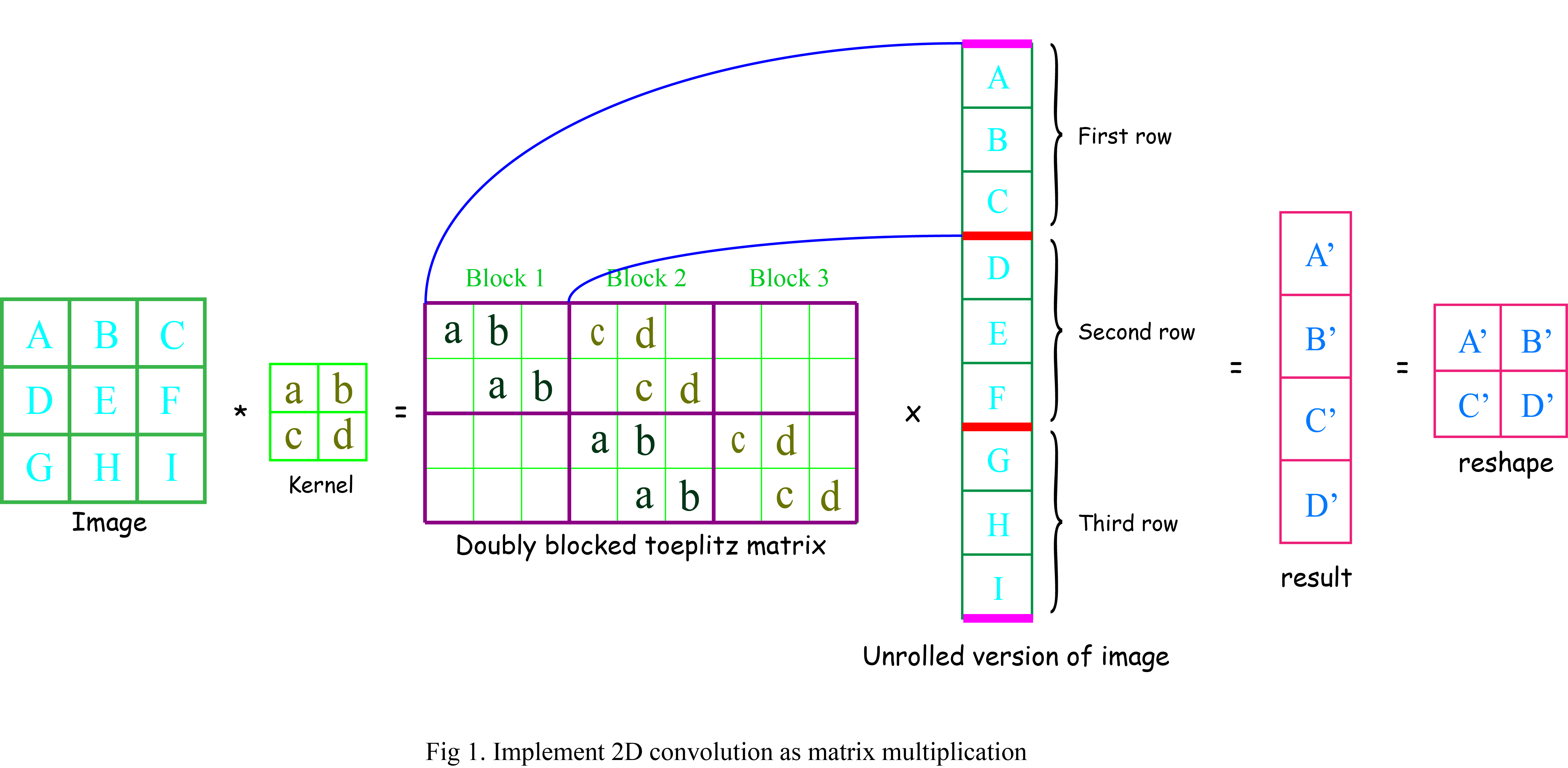从图1中可以看出，将卷积运算转化为矩阵乘积运算时，卷积核所导出的矩阵是一个双重块状toeplitz矩阵，称为卷积矩阵。卷积矩阵之所以叫双重块状toeplitz矩阵，是因为组成该矩阵的每一个分块矩阵都是toeplitz矩阵，且如果将每一个分块矩阵看做一个“元素”，那么由分块矩阵元素组成的大矩阵也是一个toeplitz矩阵。这样，如果我们将影像按照行拉伸成一维向量，按照矩阵乘法就可以发现，卷积核与原始影像的卷积就是卷积矩阵与拉伸影像的乘积的拉伸。在对结果进行reshape之后，就得到卷积之后的二维影像。

以上过程定义的卷积称为正常的卷积。正常卷积之所以正常，是因为定义输入影像的维度大于输出影像的维度。这也是最直观的结果，因为在valid卷积的情况下，只有卷积核完全覆盖原始图像的地方才能执行卷积的操作，因此必然会导致影像大小的缩小。图1.定义的正常卷积利用2x2卷积核将一个3x3的影像卷积为2x2的矩阵结果。在执行该卷积时将2x2的卷积核改写成一个4x9的双重toeplitz矩阵，而影像可以拉伸成一个9x1的向量，这样，用4x9的卷积矩阵去乘一个9x1的影像向量就得到一个4x1的结果影像的拉伸，然后将这个拉伸的影像重新排列成2x2的矩阵就是卷积的结果，而且这个结果与直接将2x2的卷积核利用卷积的定义与原始影像进行卷积的结果相同。

而转置卷积考虑的问题是，定义一个2x2的原始影像，给定一个2x2的卷积核，能不能将这个较小的原始影像转换成一个较大的3x3的结果呢？当然可以！考虑本小节的转置卷积问题，卷积核可以按图1写成同样的4x9的双重toeplitz矩阵。如果我们该双重toeplitz矩阵转置成9x4的矩阵，然后再将2x2的较小原始影像拉伸成4x1的长条，这样，这两个矩阵的乘积是一个9x1的矩阵，接着将这个矩阵重新排列成3x3，不就是我们想要的从小到大的结果吗？这其实就是转置卷积的所进行的操作！因此转置卷积中“转置”的第一层意思，就是将一个卷积核导出双重toeplitz矩阵转置，然后乘以小影像的拉伸并reshape，得到大影像结果（图2）。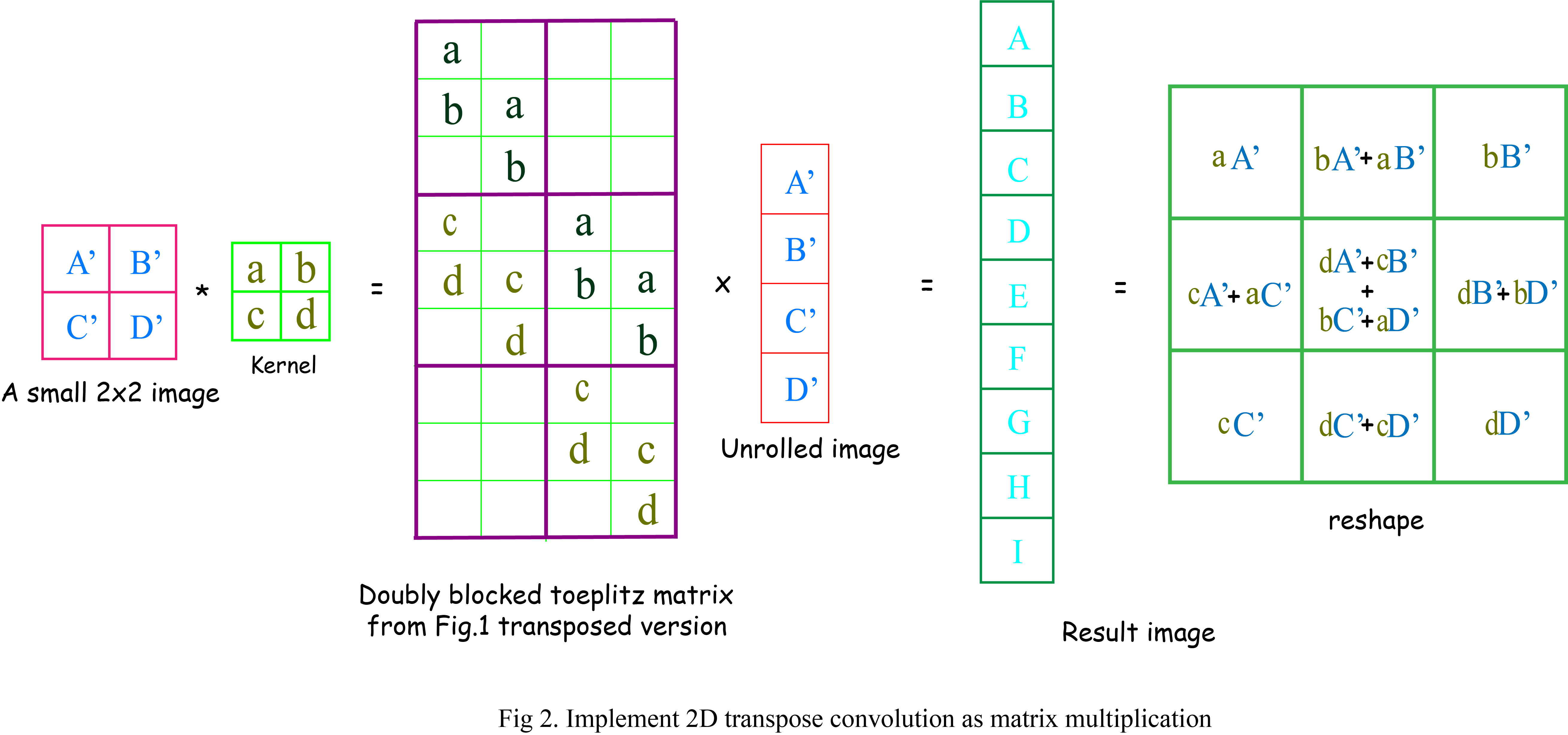在图2中可以很明显的看出，卷积核导出的卷积矩阵正是图1中双重toeplitz矩阵的转置，利用这个矩阵乘以展开后的2x2的影像得到了一个9x1的一维影像，将其重新排列成3x3，就是了转置卷积的结果。细心的同学可能注意到了我之前提到，转置双重toeplitz矩阵仅仅是转置卷积的第一层意思，而且在图2中我们显式的将矩阵乘积的结果写了出来。看看3x3的结果矩阵中间那一项[dA' + cB' + bA' + aD']想到了什么？没错，这不就是原始2x2卷积核翻转之后与2x2影像的卷积吗？那四周其余的项是怎么来的呢？一个合理的猜测是将原始2x2的影像在边上补0之后利用翻转的卷积核卷积得到的，而这个猜测是完全正确的。所以这里我给出转置卷积的第二重意思，即将原始较小的影像周围补0，然后利用翻转的卷积核对补0后的影像进行卷积，即为转置卷积的结果（图3）。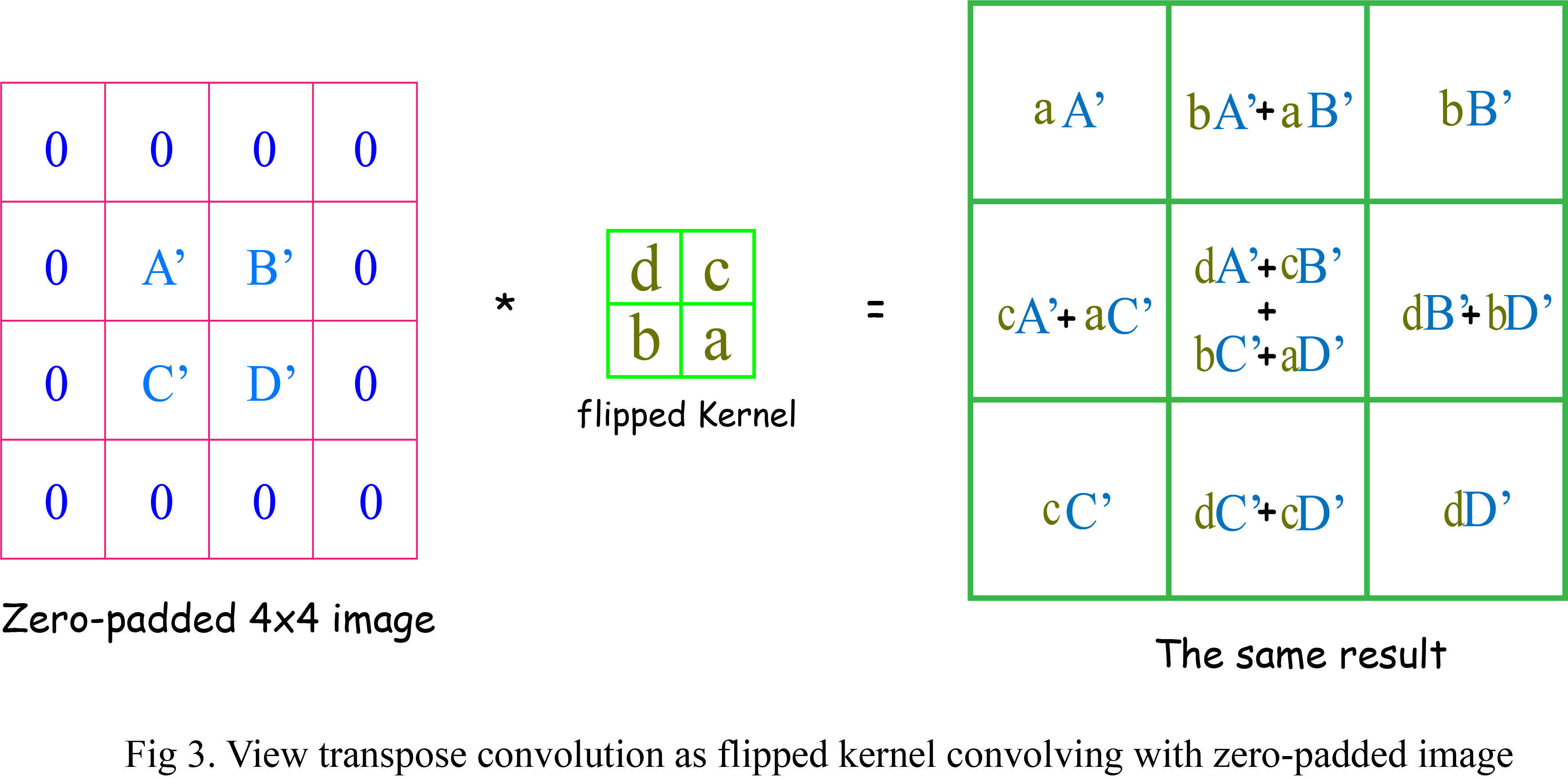从图3中可以看出，对原始影像周围进行补0，然后将卷积核上下，左右翻转，进行常规的卷积操作，可以的到和图2将toeplitz矩阵转置后进行矩阵乘积得到一样的结果。为了让大家理解更充分，我写了一段将卷积转换为乘积的python代码，因为没有用稀疏表示，因此效率不高，权当加强理解使用，大家可以随意下载执行。

#copyright: Arieslady

import numpy as np

def create_doubly_block_toeplitz_matrix(block_matrix_list, num_of_moving):
'''
block_matrix_list is the list of sub-matrices that form doubly block toeplitz matrix
num_of_moving is the times of moving sub-matrices along the row
return the created doubly block toeplitz matrix

example:
block_matrix_list = [
[[1. 2. 0.]
[0. 1. 2.]],
[[3. 4. 0.]
[0. 3. 4.]]
]
num_of_moving = 2

result:
[[1. 2. 0. 3. 4. 0. 0. 0. 0.]
[0. 1. 2. 0. 3. 4. 0. 0. 0.]
[0. 0. 0. 1. 2. 0. 3. 4. 0.]
[0. 0. 0. 0. 1. 2. 0. 3. 4.]]
'''
unit_height, unit_width = block_matrix_list.shape
num_of_units = len(block_matrix_list)

new_height = num_of_moving * unit_height
new_width = (num_of_moving + 1) * unit_width

moving_mat = np.concatenate(block_matrix_list,axis=1)

dtype = moving_mat.dtype
new_mat_block_row_list = []

for i in range(num_of_moving):
left_zero_mat = np.zeros((unit_height,i * unit_width),dtype=dtype)
right_zero_mat = np.zeros((unit_height, (num_of_moving - 1 - i) * unit_width),dtype=dtype)
new_mat_block_row_list.append(np.concatenate((left_zero_mat, moving_mat, right_zero_mat),axis=1))

return np.concatenate(new_mat_block_row_list,axis=0)

def create_convolution_matrix(input_image, kernel):
'''
create convolution matrix from input_image and kernel
'''

img_height,img_width = input_image.shape
k_size = kernel.shape

block_matrix_height = (img_height - k_size + 1)
block_matrix_width  =  img_width
block_matrix_list = []

dtype = kernel.dtype
for i in range(k_size):
block_matrix = np.zeros((block_matrix_height, block_matrix_width),dtype=dtype)
for j in range(block_matrix_height):
block_matrix[j,j:j+k_size] = kernel[i,:]
block_matrix_list.append(block_matrix)

num_of_moving = img_height - 1

return create_doubly_block_toeplitz_matrix(block_matrix_list, num_of_moving)

def conv_as_matmul(image, kernel):
'''
use matrix multiplication to conduct convolution
'''
img_height,img_width = image.shape
k_size = kernel.shape
new_height = img_height-k_size+1
new_width = img_width-k_size+1

conv_mat = create_convolution_matrix(image, kernel)
image_flatten = image.reshape(image.size,1)
return np.matmul(conv_mat, image_flatten).reshape(new_height, new_width)

if __name__ == '__main__':

#simulated image and kernel
input_image = np.arange(9,dtype=np.float32).reshape(3,3) + 1
kernel = np.arange(4,dtype=np.float32).reshape(2,2) + 1

#show image and kernel
print('Image:\n{0}'.format(input_image))
print('Kernel:\n{0}'.format(kernel))

#create conv matrix given image and kernel
conv_mat = create_convolution_matrix(input_image,kernel)
print('Convolutional matrix induced from kernel:\n{0}'.format(conv_mat))

#do convolution with conv_mat
conv_result = conv_as_matmul(input_image, kernel)
print('Convolution result:\n{0}'.format(conv_result))


展开全文• @图像处理入门学习知识（一） ...我们可以运用有关循环矩阵或Toepliz矩阵的特点来构造卷积矩阵。本文将介绍该转换过程及部分matlab实现代码。 一维图像离散降质模型 在一维离散系统中，设输入信号f(x)加性噪...
@图像处理入门学习知识（一）
卷积算子的矩阵向量乘积表示&一维离散降质模型
在图像处理过程中，有时候我们需要将卷积运算转化为矩阵乘积运算，这也是离散降质模型构成的基础，那么怎么将卷积乘法转化为矩阵向量乘法呢 。我们可以运用有关循环矩阵或Toepliz矩阵的特点来构造卷积矩阵。本文先介绍一维离散降质模型转换过程及部分代码。
一维图像离散降质模型
在一维离散系统中，设输入信号f(x)与加性噪声n(x)长度为A, h(x)为B点长度的冲激响应函数，为了避免各个周期重叠，将f(x)、n(x)、h(x)补零延拓至长度N≥A+B-1, 得到fe(x)、ne(x)、he(x)，可表示为:
$\begin{array}{l} {\rm{f}}_{\rm{e}} {\rm{(x) = }}\left\{ \begin{array}{l} {\rm{f(x),0}} \le {\rm{x}} \le {\rm{A - 1}} \\ {\rm{0,A}} \le {\rm{x}} \le N{\rm{ - 1}} \\ \end{array} (1.1.1) \right. \\ h_{\rm{e}} {\rm{(x) = }}\left\{ \begin{array}{l} {\rm{h(x),0}} \le {\rm{x}} \le B{\rm{ - 1}} \\ {\rm{0,B}} \le {\rm{x}} \le N{\rm{ - 1}} \\ \end{array}(1.1.2) \right. \\ n_{\rm{e}} {\rm{(x) = }}\left\{ \begin{array}{l} {\rm{n(x),0}} \le {\rm{x}} \le {\rm{A - 1}} \\ {\rm{0,A}} \le {\rm{x}} \le N{\rm{ - 1}} \\ \end{array}(1.1.3) \right. \\ \end{array}$
根据卷积定理可得，一维离散降质模型用离散卷积形式表示为：
$g_{\rm{e}} (x) = {\rm{f}}_{\rm{e}} (x) * h_{\rm{e}} (x) + n_{\rm{e}} (x) = \sum\limits_0^{N - 1} {f_{\rm{e}} (n)} f_{\rm{e}} (x - n) + n_{\rm{e}} (x) (1.1.4)$式中，x=0,1,2,···，N-1。将上式(1.1.4)卷积形式用矩阵向量表示为：
${\rm{\bf{g} = \bf{Hf} + \bf{n}}}$
卷积矩阵$\bf{H}$为$h_e(x)$的矩阵形式， $\bf{H}$为循环矩阵，上式中，$\bf{g}$、$\bf{f}$、$\bf{n}$均为N维列向量，如下：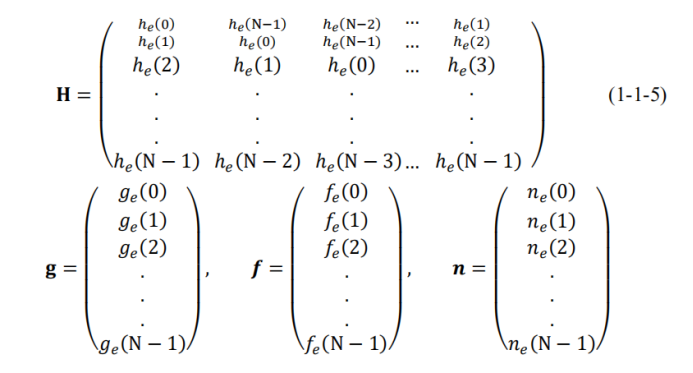若不添加噪声项n进行测试，此时则有${\bf{Hf}=f(x)*h(x)}$，$“*”$代表卷积运算，结果如下：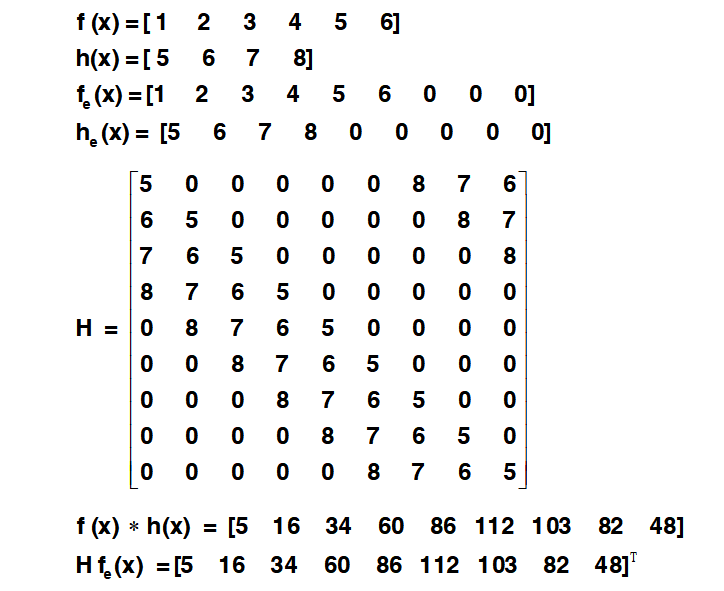matlab代码：

function [M,X] = TMtx1(x,h)%一维离散降质模型
[m1,n1]=size(x); %n1为x的元素个数
[m2,n2]=size(h); %n2为h的元素个数
N=n1+n2-1;       %补零延拓，避免周期重叠产生误差
x(N)=0;
x(N)=0;
h(N)=0;
x1=x;
h1=h;
% 构建延拓矩阵 N
x2 = x1(:);
h2 = h1(:);
%% 循环矩阵方法一
t=zeros(1,N);
t(1)=h2(1);
t(1,2:N)=h2(N:-1:2);
M = toeplitz(h2,t);
X = x1(:);
%% 方法二 循环矩阵
% A=zeros(N,N);
% A(1,1)=h2(1);
% A(1,2:N)=h2(N:-1:2);
%第一行
%  for i=2:N            %i为行数
%      A(i,1)=A(i-1,N);  %第i行的第一列与最后一列的关系
%      A(i,2:N) =A(i-1,1:N-1); % 第i行的第其他列与上一行的关系
%  end
end




展开全文图像处理
• 矩阵乘法，结果矩阵每个元素是等长度一行一列向量乘再累加求结果。 因此核心的矩阵算法如下： float test(float* a, float* b, int n) { float c = 0; for(int i=0; i&lt;n; i++) { c += (*a...
卷积可以通过im2col转化为矩阵乘。

而矩阵乘法，结果矩阵中的每个元素是等长度的一行与一列向量乘再累加求结果。

因此核心的矩阵算法如下：

float test(float* a, float* b, int n)
{
float c = 0;
for(int i=0; i<n; i++)
{
c += (*a++) * (*b++);
}
return c;
}

这段代码，如果手工优化，无非是循环展开等等...不过现代编译器的优化了得，在使用-ffast-math和-ftree-vectorize优化选项后，编译器也能做到循环展开和向量化（使用SIMD）指令，如下是上述代码用g++ -S 生成的汇编代码

_Z4testPfS_i:
subs	w8, w2, #1  // w8 = n-1, if w8 < 0, return 0
b.lt	.LBB0_3

add	x8, x8, #1 // x8+1
cmp		x8, #7
fmov	s0, wzr
b.hi	.LBB0_4 // 如果数量超过8个，走循环

mov	 w8, wzr
b	.LBB0_8 // 跳转到尾巴处, 处理尾巴

.LBB0_3:
mov	 w0, wzr
ret

.LBB0_4:
and	w9, w2, #0x7    // w9 = w2 & 0x7, 循环展开
sub	x8, x8, x9 // 计算i的下标
cbz	x8, .LBB0_8

lsl	x13, x8, #2 // 根据i的下标计算偏移量，x13存放的是float的地址偏移量 左移2位，因为float是4B
add	x10, x1, #16 // x1是b的地址, 不明白+16是何用意，x10是本次首地址
add	x11, x0, #16 // x0是a的地址, 不明白+16是何用意，x11是本次首地址
movi v0.2d, #0000000000000000 // 2个long等于4个float，赋值为0

mov	 x12, x8

add	 x0, x0, x13 // a的下一个地址
add	 x1, x1, x13 // b的下一个地址
movi v1.2d, #0000000000000000 // 2个long等于4个float，赋值为0

.LBB0_6:
ldp	q2, q3, [x11, #-16] // 从x11-16的地址取8个float，放在q2和q3 中
ldp	q4, q5, [x10, #-16] // 从x11-16的地址取8个float，放在q2和q3 中

add	x10, x10, #32           // =32 // x10向上走8个float
sub	x12, x12, #8            // =8 // 下标i减8
add	x11, x11, #32           // =32 // x11向上走8个float
fmla	v0.4s, v2.4s, v4.4s // 0 = 2 + 4 + 0，向量累加
fmla	v1.4s, v3.4s, v5.4s // 1 = 3 + 5 + 1，向量累加

cbnz	x12, .LBB0_6 // 判断循环是否到0

ext	v1.16b, v0.16b, v0.16b, #8

dup	v1.4s, v0.s

cbz	w9, .LBB0_10

.LBB0_8:
sub		w8, w2, w8

.LBB0_9:
ldr	s1, [x0], #4 // 不足8个的，还剩一点尾巴，1个1个的取
ldr	s2, [x1], #4
sub	w8, w8, #1  // i下标1个1个处理
fmadd	s0, s2, s1, s0  // 0 = 2 + 1 + 0, 单个float相加
cbnz	w8, .LBB0_9

.LBB0_10:
fcvtzs	w0, s0
ret



展开全文ARM NEON
• 编码器与解码器神经网络本质上就是一个线性变换，通过将输入多维向量与权重矩阵相乘，得到一个新多维向量。当输入向量维度高于输出向量维度时，神经网络就相当于一个编码器，实现了对高维向量低维特征
• 1的映射关系时，保持了输出相对于input中的位置性关系对核矩阵做以下变形：卷积核的滑动步骤变成了卷积矩阵的扩增卷积的矩阵乘法变成以下形式：核矩阵重排，输入featuremap变形为向量卷积(1---->多 的映射)...
• 深度学习中的卷积与转置卷积1 卷积2 转置卷积（反卷积）3 个人理解 1 卷积 输入平展为 16×1 的矩阵，并将卷积核转换为一个稀疏矩阵C（4×16）。然后，在稀疏矩阵和平展的输入之间使用矩阵乘法。之后，再将所得到的...深度学习 神经网络 计算机视觉
• 哈达马积（Hadamard product） 两个矩阵对应元素乘积 * 卷积操作一般就是这个符号 克罗内克积（Kronecker Product）克罗内克积也被称为直积或张量积 x外面一个圈 https://baike.baidu.com/item/%E7%9F%A9%E9%98%
• 卷积尺寸为 3x3，其元素矩阵为： 正向卷积操作：步长 strides = 1, 填充 padding = 0,输出形状为 2x2，该过程如下图所示： 在上面这幅图中，底端为输入，上端为输出，卷积核为 3x3。如果我们用矩阵乘法...深度学习
• Caffe中的卷积计算是将卷积核矩阵和输入图像矩阵变换为两个大的矩阵AB，然后AB进行矩阵相乘得到结果C（利用GPU进行矩阵相乘的高效性），三个矩阵的说明如下： （1）在矩阵A中  M为卷积核个数，K=k*k，...caffe
• 1.piano_roll向量v 这是一个二维矩阵，两个轴分别为时间和音高，对应数值表示力度。它把各个乐器音都合成在一起了。可以调整参数是fs：扫描频率。默认是fs=100，表示对于midi音频每秒扫描100次。为了在...
• （1）首先将卷积感知野的乘积转换一下，将4*4的卷积核转换为下面的矩阵，然后4*4的原始数据转换为16*1的向量，这样子下面的矩阵一共有四行对应了四次卷积运算，0则表示在实际卷积过程中，卷积核原图不重叠的位置...
• 我们说 deconvolution 反卷积 transposed convolution 转置卷积 fractional strided convolution 都是同一个概念 ...大家可能对于反卷积的认识有一个误区，以为通过反卷积...向量矩阵做点积 卷积的正向传...
• BP 人工神经网络是以一维向量的方式进行输入，而卷积神经网络以二维矩阵格式数据进行输入，其网络各层都是二维阵列形式处理数据，这样形式正好符合数字图像二维矩阵格式，图像以二维矩阵输入正...深度学习
• 卷积棋盘格模式最近需要通过反卷积生成双目估计深度图,在阅读反卷积的相关资料时候看到了Deconvolution and Checkerboard Artifacts,对反卷积(转置卷积)和反卷积生成棋盘... 输入矩阵展开为16维向量,x 输出
• 一.全连接层： 全连接层需要把输入拉成一个列项向量 ,如下...这时候，再乘一个权重，这个权重要把12个像素点都包含进去，所以这个权重的矩阵形式应该是1X12,所以经过一个全连接层后输出就是1X12X12X1=1X1，这时候需要神经网络 网络 深度学习
• (离散)卷积操作其实是仿射变换的一种: 对输入向量进行线性变换, 再加一个bias. 是一种线性变换. 它本身也满足线性函数的... 则定义在它们之上的卷积操作可以写为矩阵$$C$$与向量$$x$$的乘法. $$C^T$$为: $$C$$定义...
• 编码器与解码器神经网络本质上就是一个线性变换，通过将输入多维向量与权重矩阵相乘，得到一个新多维向量。当输入向量维度高于输出向量维度时，神经网络就相当于一个编码器，实现了对...
• 最后，将转换后向量整数标签转换为二进制矩阵，以促进kerasto_categorical类进行分类交叉熵。 神经网络拓扑和结果摘要 二进制交叉熵损失函数Rmsprop优化器一起用于此分类任务。 经过10个时间段后，训练和...Python
• 简单的卷积就不说了，向量卷积用此函数用conv效果相同，矩阵卷积用此函数conv2的二维卷积效果相同。此函数的方便之处在于支持三维卷积：其实相对于conv2来说就是省了一个for循环。对于三维卷积，比如A矩阵大小为...
• 向量与列向量意义 4 损失函数与梯度 4 激活单元缺陷 5 卷积层算法理解 6 为什么要引入卷积 6 一些超级参数 6 接入神经网络 7 部分具体实现 7 大体步骤 7 关于卷积运层反向传播的的一些探讨 7 化卷积...神经网络
• 如上图所示,把待卷积的卷积核展开成行向量,把待卷积的图像块展开成列向量,二者相乘即可得到卷积后的结果. 例如，如果大小为[227x227x3]步幅4和填充0的输入11x11x3滤波器进行卷积，那么我们将在输入中采样[11x11x3]...
• 卷积模型是Conv2D层和MaxPooling2D层堆叠，本例中卷积神经网络处理大小为（28，28，1）输入张量，这正是MNIST图像格式 建立分类卷积模型 模型建立训练 设置训练容器 优化算法、损失函数、监督矩阵...
• 卷积运算，两个输入张量(输入数据和卷积核)进行卷积，输出代表来自每个输入信息张量。 tf.nn.conv2d完成卷积运算。 卷积核(kernel)，权值、滤波器、卷积矩阵或模版，filter。 权值训练习得。 卷积核(filter参数)...
• GEMM矩阵相乘深度学习1. GEMM矩阵相乘1.1 GEMM算法基础... GEMM与卷积计算2.1 计算卷积的方法2.2 Img2col2.2.1 CNN中张量存储2.2.2 卷积运算转化为GEMM 1. GEMM矩阵相乘 1.1 GEMM算法基础   GEMM（General Matrix...